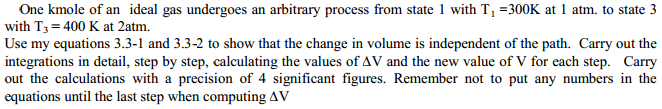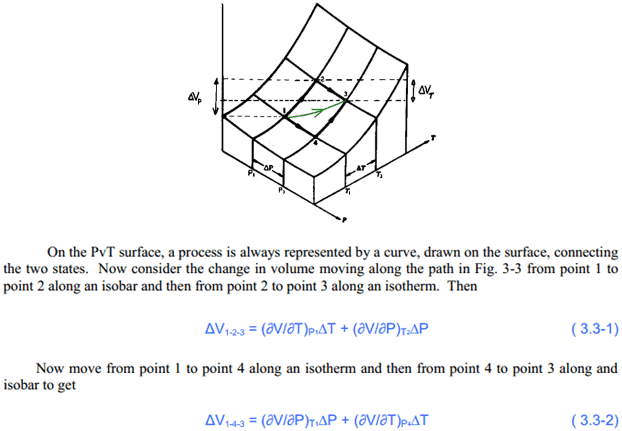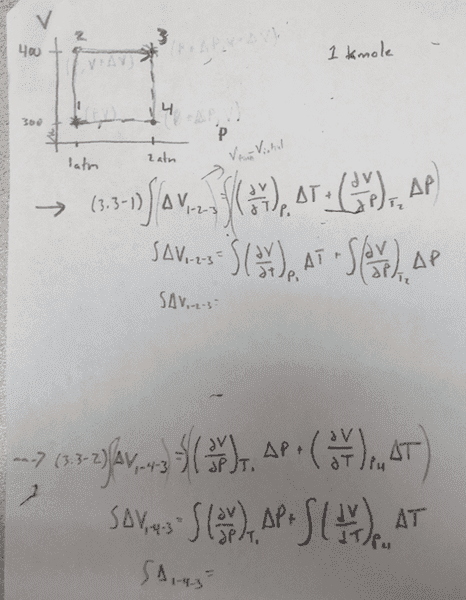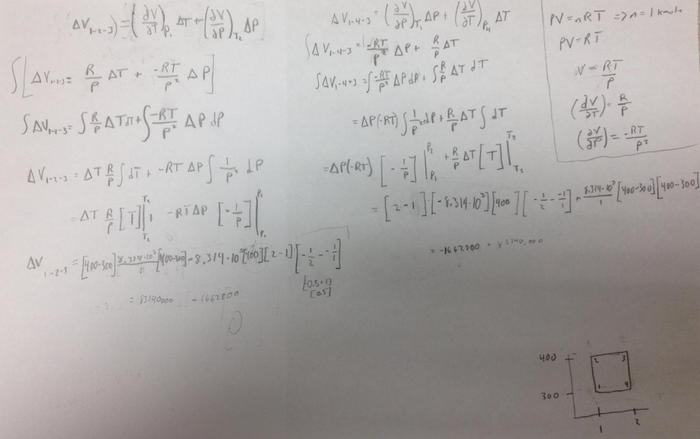# Show that the Change in Volume is Independent of the Path

## Homework Statement## Homework Equations## The Attempt at a SolutionI understand what the question is asking. Both ways I should get the same answer. I'm having trouble figuring out the mathematics behind this question.

Hi.
You're dealing with an ideal gas: what's the equation of state? (the one relating P, V and T)

Hi.
You're dealing with an ideal gas: what's the equation of state? (the one relating P, V and T)

The equation of state would be PV=nRT.
P- pressure
V- volume
n- number of moles
T-temperature
R-universal constant

That was your only missing piece, now you can work the integrals.

That was your only missing piece, now you can work the integrals.

So would I have to change the equation of state around and plug it into each change of V, change of T, and change of P?

Edit: I should plug them into the partial difference equation? The change in should be constants I think.

Edit(again): The only equation I really have to plug in is V=(RT)/P. I think I'm just taking different differentials followed by integration.

Last edited:
Use the equation of state to determine the derivatives. Example:
PV = nRT
so that, if n is constant:
VΔP + PΔV = nRΔT
When P is constant: $\frac{\partial V}{\partial T}$ = $\frac{nR}{P}$,
When T is constant: $\frac{\partial V}{\partial P}$ = – $\frac{nRT}{P^{2}}$

Just pay attention to what's constant and what's not when following your equations. Take the time to understand what you're doing, but you have everything you need.

Use the equation of state to determine the derivatives. Example:
PV = nRT
so that, if n is constant:
VΔP + PΔV = nRΔT
When P is constant: $\frac{\partial V}{\partial T}$ = $\frac{nR}{P}$,
When T is constant: $\frac{\partial V}{\partial P}$ = – $\frac{nRT}{P^{2}}$

Just pay attention to what's constant and what's not when following your equations. Take the time to understand what you're doing, but you have everything you need.

Here is the work I have done so far. I did use the equations but I was having a hard time understanding in my mind the final values to plug in specifically for the final T value. I assumed it was referring to the final state so I plugged in 400 K. Please let me know if I made any mistakes.
Thanks again for the help.Ok, you have to be careful when integrating. First with:
ΔV1-2-3 = ($\frac{\partial V}{\partial T}$)P1 ΔT + ($\frac{\partial V}{\partial P}$)T2 ΔP,
You get:
∫ΔV1-2-3 = Vfinal – Vinitial = ∫($\frac{\partial V}{\partial T}$)P1 ΔT +∫($\frac{\partial V}{\partial P}$)T2 ΔP = [V(P1,T2) – V(P1,T1)] + [V(T2,P2) – V(T2,P1)]
= R($\frac{ΔT}{P_{1}}$ + $\frac{T_{2}}{P_{2}}$ – $\frac{T_{2}}{P_{1}}$)

So you see that using integrations is purely formal here: at the end you don't have to differentiate or integrate anything.
Also, be careful with your units: atm is not a S.I. unit, so the gas constant must be adjusted if you use atms...

Ok, you have to be careful when integrating. First with:
ΔV1-2-3 = ($\frac{\partial V}{\partial T}$)P1 ΔT + ($\frac{\partial V}{\partial P}$)T2 ΔP,
You get:
∫ΔV1-2-3 = Vfinal – Vinitial = ∫($\frac{\partial V}{\partial T}$)P1 ΔT +∫($\frac{\partial V}{\partial P}$)T2 ΔP = [V(P1,T2) – V(P1,T1)] + [V(T2,P2) – V(T2,P1)]
= R($\frac{ΔT}{P_{1}}$ + $\frac{T_{2}}{P_{2}}$ – $\frac{T_{2}}{P_{1}}$)

So you see that using integrations is purely formal here: at the end you don't have to differentiate or integrate anything.
Also, be careful with your units: atm is not a S.I. unit, so the gas constant must be adjusted if you use atms...

Ok thanks I'm going to take another look at what I did. Will post when I finish.